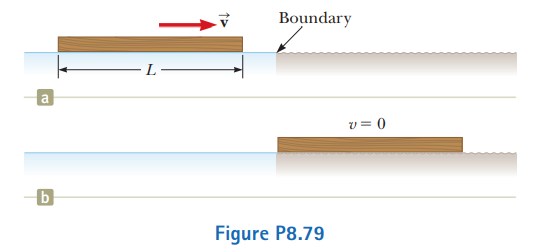# Problem: A uniform board of length L is sliding along a smooth, frictionless, horizontal plane as shown in Figure P8.79a. The board then slides across the boundary with a rough horizontal surface. The coefficient of kinetic friction between the board and the second surface is µk. (a) Find the acceleration of the board at the moment its front end has traveled a distance x beyond the boundary. (b) The board stops at the moment its back end reaches the boundary as shown in Figure P8.79b. Find the initial speed v of the board.

⚠️Our tutors found the solution shown to be helpful for the problem you're searching for. We don't have the exact solution yet.

###### Problem Details

A uniform board of length L is sliding along a smooth, frictionless, horizontal plane as shown in Figure P8.79a. The board then slides across the boundary with a rough horizontal surface. The coefficient of kinetic friction between the board and the second surface is µk. (a) Find the acceleration of the board at the moment its front end has traveled a distance x beyond the boundary. (b) The board stops at the moment its back end reaches the boundary as shown in Figure P8.79b. Find the initial speed v of the board.